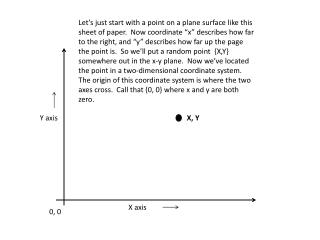Download PresentationX, Y# X, Y - PowerPoint PPT Presentation

Download Presentation##### X, Y

Download Policy: Content on the Website is provided to you AS IS for your information and personal use and may not be sold / licensed / shared on other websites without getting consent from its author. While downloading, if for some reason you are not able to download a presentation, the publisher may have deleted the file from their server.

- - - - - - - - - - - - - - - - - - - - - - - - - - - E N D - - - - - - - - - - - - - - - - - - - - - - - - - - -
##### Presentation Transcript

1. Let’s just start with a point on a plane surface like this sheet of paper. Now coordinate “x” describes how far to the right, and “y” describes how far up the page the point is. So we’ll put a random point {X,Y} somewhere out in the x-y plane. Now we’ve located the point in a two-dimensional coordinate system. The origin of this coordinate system is where the two axes cross. Call that {0, 0} where x and y are both zero. Y axis X, Y X axis 0, 0

2. You could also draw a straight arrow from the origin of the coordinate system out to that point. The arrow representation of that point {X,Y} is a vector. y q x

3. This is interesting because now the point we started with, which has become a vector, looks like a right triangle with side lengths (a) and (b), and hypotenuse (c). So the length of the hypotenuse is also the length of the vector and from trigonometry: Cos(q)= x/c and Sin(q)= y/c y (c) (b) q (a) x

4. Introduction to the “DOT product” The dot product is a beautiful and useful generalization of normal multiplication - only now you multiply vectors which are a bit more complicated . Since this is a new kind of multiplication, lets also give it a new symbol • Lets call our vector from the last page just V for short. V really contains the two numbers x and y. The length of V squared is x2+y2 or x*x+y*y from the Pythagorean formula. In words : “V squared, or V dot V, or V•V, equals the length of the vector squared, and also equals the x component of V times the x component of V, plus the y component of Vtimes the y component of V. Nothing new yet- remember this is a warm-up.

5. Now expand the discussion to include two vectors V1 and V2 The dot product V1•V2 equals the x component of V1 times the x component of V2 plus the y component of V1 times the y component of V2. In symbols: V1•V2 =x1*x2+y1*y2 Now this is becoming interesting. y2 y1 f q x2 x1

6. Now lets assemble the pieces of that dot product ! Remember from trigonometry that the cosine of an angle equals the length of the adjacent side of a right triangle divided by the hypotenuse. The two hypotenuse lengths are just the lengths of the two vector V1 and V2. So…. x1*x2= cos(q)*cos(f)*length(V1)*length(V2) The same argument works for sines, so y1*y2= sin(q)*sin(f)*length(V1)*length(V2) Putting these piece together gives: V1•V2=length(V1)*length(V2)*(cos(q)*cos(f)+sin(q)*sin(f)) If you remember the easy derivations of the angle addition formulae from tidbit 1, this comes to: V1•V2=length(V1)*length(V2)*cos(q-f). The angle difference (q-f) is just the angle included between the two vectors. So this is one of several useful applications of the dot product – finding the angle between two vectors.

7. A 3D example – tetrahedral bonds. A molecule of methane (CH4) is sketched out below. The four hydrogens are colored red and the central carbon in black. If the four bonds are symmetrical, the hydrogens should occupy opposite corners of a cube. Lets find the central angle between any pair of bonds y x 0, 0, 0 z

8. Center the cube so that the carbon sits at the origin of the 3 dimensional coordinate system. Since the actual size of the cube won’t matter, lets just give it a side length of 2. Then the vector from the origin to the top left hydrogen ends at x = -1, y = 1, z = -1. So V1 = {-1, 1, -1}. Doing the same for the hydrogen at the top right gives us V2={1, 1, 1}. As before V1•V2=x1*x2+y1*y2+z1*z2. Plugging the numbers in gives us V1•V2= -1. This also equals length(V1)*length(V2)*cos(q). The result is cos(q)= -1/3 so q (the angle between bonds) is about 109.5 degrees. The vector lengths simplify since the two are equal. That leads to length2 = V1•V1 = 3 y x z